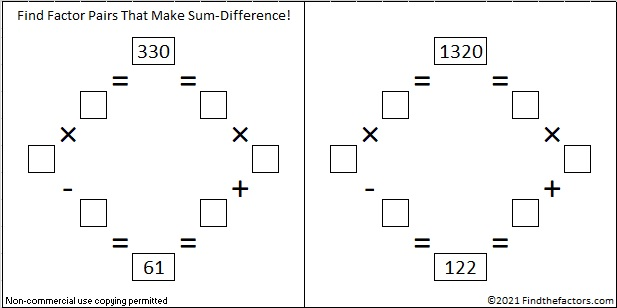# 1320 Christmas Factor Trees

Contents

### Today’s Puzzle:

Regardless of its size, an evergreen tree is a mighty symbol at Christmastime. Today’s factoring puzzle features a couple of relatively small Christmas trees, but don’t think for even one minute that these little trees make for an easy puzzle. It’s a level 6 puzzle so there are several places that the clues might trick you. Use logic throughout the entire process, and you should be able to solve it!

Print the puzzles or type the solution in this excel file: 12 factors 1311-1319

### Factor Trees for 1320:

That factoring puzzle has nothing to do with the factors of 1320. In case you are looking for factor trees for the number 1320, here are a few of the MANY possible ones:

### Factors of 1320:

• 1320 is a composite number.
• Prime factorization: 1320 = 2 × 2 × 2 × 3 × 5 × 11, which can be written 1320 = 2³ × 3 × 5 × 11.
• The exponents in the prime factorization are 3, 1, 1, and 1. Adding one to each and multiplying we get (3 + 1)(1 + 1)(1 + 1) )(1 + 1) = 4 × 2 × 2 × 2 = 32. Therefore 1320 has exactly 32 factors.
• Factors of 1320: 1, 2, 3, 4, 5, 6, 8, 10, 11, 12, 15, 20, 22, 24, 30, 33, 40, 44, 55, 60, 66, 88, 110, 120, 132, 165, 220, 264, 330, 440, 660, 1320
• Factor pairs: 1320 = 1 × 1320, 2 × 660, 3 × 440, 4 × 330, 5 × 264, 6 × 220, 8 × 165, 10 × 132, 11 × 120, 12 × 110, 15 × 88, 20 × 66, 22 × 60, 24 × 55, 30 × 44 or 33 × 40
• Taking the factor pair with the largest square number factor, we get √1320 = (√4)(√330) = 2√330 ≈ 36.331804

### Sum-Difference Puzzles:

330 has eight factor pairs. One of those pairs adds up to 61, and  another one subtracts to 61. Put the factors in the appropriate boxes in the first puzzle.

1320 has sixteen factor pairs. One of the factor pairs adds up to ­122, and a different one subtracts to 122. If you can identify those factor pairs, then you can solve the second puzzle!The second puzzle is really just the first puzzle in disguise. Why would I say that?

1320 is the third number that is at the top of more than one Sum-Difference Puzzle. This next one is a primitive.

Again, 1320 has sixteen factor pairs. One of the factor pairs adds up to 73, and a different one subtracts to 73. If you can identify those factor pairs, then you can solve this puzzle!If you would like a little help finding those factor pairs that make sum-difference, the chart below lists all of 1320’s factor pairs with their sums and their differences.### More about the Number 1320:

1320 is the sum of consecutive primes FOUR different ways:
It is the sum of the eighteen primes from 37 to 109,
107 + 109 + 113 + 127 + 131 + 137 + 139 + 149 + 151 + 157 = 1320,
149 + 151 + 157 + 163 + 167 + 173 + 179 + 181 = 1320, and
659 + 661 = 1320.

1320 is the hypotenuse of a Pythagorean triple:
792-1056-1320 which is (3-4-5) times 264.

This site uses Akismet to reduce spam. Learn how your comment data is processed.